ISEE Upper Level Math : How to find mode

Example Questions

Example Question #11 : Mode

What is the mode of the following set of numbers?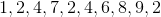Explanation:

To determine the mode, you need to identify which number appears most frequently in the set of numbers.

In this set of numbers, the number 2 shows up three times, and no other number occurs three or more times. Thus, it is the only mode.

Example Question #12 : Mode

What is the mode value in the set below?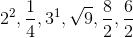Explanation:

The first step is to convert the expressions in the setto their simplified forms. This gives us: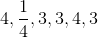While the value of 4 appears twice, given that the value 3 appears 3 times, it is the mode in this set.

Example Question #13 : Mode

Find the mode of the following data set: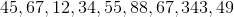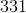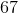Explanation:

Find the mode of the following data set:Begin by putting your numbers in increasing order: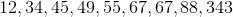We can identify the mode simply by finding the most common term.

In this data set, we have two 67's. There are no other duplicate numbers, so we have our answer: 67

Example Question #14 : Mode

Find the mode of the following data set: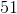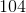Explanation:

Find the mode of the following data set: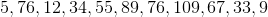Let's begin by rearranging our terms from least to greatest: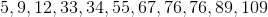Now, the mode will be our most common term.

In this case, it is 76, because there are two 76's.

Example Question #15 : Mode

Find the mode of the following data set: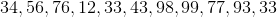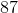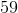Explanation:

Find the mode of the following data set:First, let's put our terms in increasing order: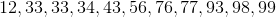Now, our mode will just be our most common term. In this case it is 33.

Example Question #16 : Mode

Find the mode of the following data set: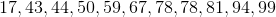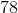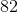Explanation:

Find the mode of the following data set:First, let's put our terms in ascending order.Next, identify the mode by choosing the most common term.

In this case, we have 2 78's. Therefore, our mode is 78.

Example Question #11 : Mode

Use the following data set to answer the question: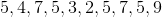Find the mode.Explanation:

To find the mode of a data set, we will find the number that occurs most often.

So, given the data setwe can see the number 5 occurs most often (it occurs 4 times.)

Therefore, the mode of the data set is 5.

Example Question #18 : Mode

Use the following data set to answer the question: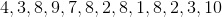Find the mode.Explanation:

To find the mode of a data set, we will find the number that occurs most often.

So, given the data setwe can see the number that occurs most often is 8 (it occurs 4 times).

Therefore, the mode of the data set is 8.

Example Question #11 : How To Find Mode

Find the mode of the following data set: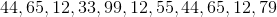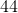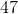Explanation:

Find the mode of the following data set:To find the mode, first put the numbers in increasing order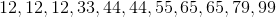Next, find the mode by simply choosing the most common number.

In this case, 12 appears three times, so it is our most common term.

Example Question #111 : Data Analysis

Use the following data set to answer the question: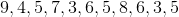Find the mode.Explanation:

To find the mode of the data set, we will find the number that appears most often. So, given the setwe can see the number that appears most often is 5 (it appears 3 times).

Therefore, the mode of the data set is 5.